• 二项式分布定义
千次阅读
2021-12-13 14:49:10

numpy 是用于数据科学计算的基础模块，其中的random模块能够非常方便的生成各种随机数据用于数据计算，本次重点记录验证概率用途的二项分布生成函数 binomial。

官网和很多帖子都详细描述了二项式分布的定义和参数解释，在这里不再单独做学术性解释了，直接做实例演示：

参数基本结构：1个试验样本，预期结果发生概率50%，试验100次：比如抛硬币

print('生成随机数为：',np.random.binomial(1, 0.5, 100))

生成随机数为： [0 1 0 0 0 1 1 0 0 0 0 0 1 1 1 0 1 0 1 0 1 0 1 1 1 1 0 0 0 1 0 0 1 1 0 0 1 0 0 0 1 1 1 0 0 1 1 1 1 1 1 0 0 1 1 1 0 1 0 1 1 0 0 1 0 0 1 0 1 0 1 1 0 1 0 1 0 1 1 1 0 0 0 1 1 0 1 0 0 1 0 0 1 0 1 0 1 1 1 1]

衍生出场景用法：当实验样本为独立样本，互相之间不影响概率的情况下，重新定义概率，进行多组实验：假定有5台试验设备，每台设备每小时发生故障的概率是10%，连续运行10小时，5台设备都完好的比例是多少？

print('生成随机数为：',np.random.binomial(5, 0.9, [5,10]))
生成随机数为： [[3 5 5 3 4 5 4 4 4 5]
[5 4 5 5 5 4 4 5 4 3]
[4 4 4 5 5 4 4 4 5 4]
[5 5 5 3 4 5 5 5 5 4]
[3 5 5 4 5 5 5 3 5 4]]

试验5组试验数据，观察数据分布，5代表5个样本都发生90%概率期望值，即全部完好状态

计算概率结果：

print (sum(np.random.binomial(5, 0.9, 10) == 5) / 10)

输出：0.6、0.7、0.6、0.5

增大试验次数至1000小时

print (sum(np.random.binomial(5, 0.9, 1000) == 5) / 1000)

多次输出结果：0.59、0.58、0.583、0.621

增大试验次数100000小时

print (sum(np.random.binomial(5, 0.9, 100000) == 5) / 100000)

多次输出结果：0.59025、0.59201、0.59158、0.58859

理论上同时完好概率应为0.9*0.9*0.9*0.9*0.9=0.59049，证明随着样本数据量上升，输出结果趋于理论实际。

反向使用，即可获得故障情况概率，取最优情况进行资源利用测算即可实现场景应用

print ('每小时内5台设备同时故障情况：',sum(np.random.binomial(5, 0.1, 100000) == 5) / 100000)
print ('每小时内4台设备同时故障情况：',sum(np.random.binomial(5, 0.1, 100000) == 4) / 100000)
print ('每小时内3台设备同时故障情况：',sum(np.random.binomial(5, 0.1, 100000) == 3) / 100000)
print ('每小时内2台设备同时故障情况：',sum(np.random.binomial(5, 0.1, 100000) == 2) / 100000)
print ('每小时内1台设备同时故障情况：',sum(np.random.binomial(5, 0.1, 100000) == 1) / 100000)
print ('每小时内5台设备均无故障情况：',sum(np.random.binomial(5, 0.1, 100000) == 0) / 100000)
输出结果：
每小时内5台设备同时故障情况： 1e-05
每小时内4台设备同时故障情况： 0.00044
每小时内3台设备同时故障情况： 0.00841
每小时内2台设备同时故障情况： 0.07306
每小时内1台设备同时故障情况： 0.32525
每小时内5台设备均无故障情况： 0.59139

注意事项：因二项式分布概率参数需满足二项式分布条件，所以概率结果应该是布尔值（ture/false）情况,最简单的场景是抛硬币的情况，其他场景应在该前提下进行应用。python
更多相关内容
• 此 m 文件返回参数为 R 和 P 的负二项式分布的偏度、峰态和峰态超量。S、K 和 E 是输入参数的大小。 语法：function [s,k,e] = nbinskekur(r,p) 输入： r - 预定义的失败次数p - 概率参数（成功的概率） 输出： s - ...matlab
• 定义 让我们来看看玩板球这个例子。假设你今天赢了一场比赛，这表示一个成功的事件。...二项式分布就是只有两个可能结果的分布，比如成功或失败、得到或者丢失、赢或败，每一次尝试成功和失败的概率相等。

## 定义

让我们来看看玩板球这个例子。假设你今天赢了一场比赛，这表示一个成功的事件。你再比了一场，但你输了。如果你今天赢了一场比赛，但这并不表示你明天肯定会赢。我们来分配一个随机变量X，用于表示赢得的次数。 X可能的值是多少呢？它可以是任意值，这取决于你掷硬币的次数。

只有两种可能的结果，成功和失败。因此，成功的概率 = 0.5，失败的概率可以很容易地计算得到：q = p – 1 = 0.5。

二项式分布就是只有两个可能结果的分布，比如成功或失败、得到或者丢失、赢或败，每一次尝试成功和失败的概率相等。

结果有可能不一定相等。如果在实验中成功的概率为0.2，则失败的概率可以很容易地计算得到 q = 1 - 0.2 = 0.8。

每一次尝试都是独立的，因为前一次投掷的结果不能决定或影响当前投掷的结果。只有两个可能的结果并且重复n次的实验叫做二项式。二项分布的参数是np，其中n是试验的总数，p是每次试验成功的概率。

在上述说明的基础上，二项式分布的属性包括：

• 每个试验都是独立的。
• 在试验中只有两个可能的结果：成功或失败。
• 总共进行了n次相同的试验。
• 所有试验成功和失败的概率是相同的。 （试验是一样的）

## 公式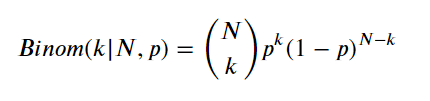𝑁⋅𝑝 表示分布的均值

• PMF( 概率质量函数 ): 是对离散随机变量的定义. 是离散随机变量在各个特定取值的概率. 该函数通俗来说,就是对于一个离散型概率事件来说, 使用这个函数来求它的各个成功事件结果的概率.

• PDF ( 概率密度函数 ): 是对连续性随机变量 的定义. 与PMF不同的是 PDF 在特定点上的值并不是该点的概率, 连续随机概率事件只能求一段区域内发生事件的概率, 通过对这段区间进行积分来求. 通俗来说, 使用这个概率密度函数 将 想要求概率的区间的临界点( 最大值和最小值)带入求积分. 就是该区间的概率.

TypeError: bar() missing 1 required positional argument: 'x'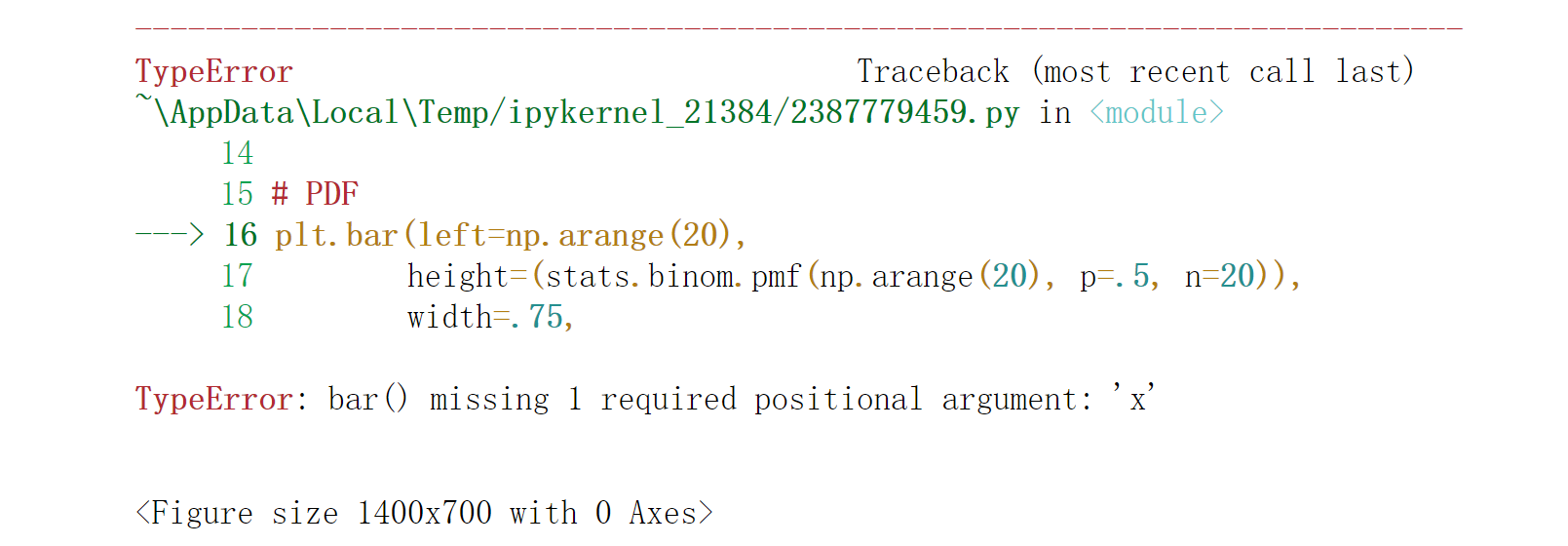将left修改为x：

plt.bar(left=np.arange(20),
height=(stats.binom.pmf(np.arange(20), p=.5, n=20)),
width=.75,
alpha=0.75
)

plt.bar(x=np.arange(20),
height=(stats.binom.pmf(np.arange(20), p=.5, n=20)),
width=.75,
alpha=0.75
)

# IMPORTS
import numpy as np
import scipy.stats as stats
import matplotlib.pyplot as plt
import matplotlib.style as style
from IPython.core.display import HTML

# PLOTTING CONFIG
%matplotlib inline
style.use('fivethirtyeight')
plt.rcParams["figure.figsize"] = (14, 7)

plt.figure(dpi=100)

# PDF
plt.bar(x=np.arange(20),
height=(stats.binom.pmf(np.arange(20), p=.5, n=20)),
width=.75,
alpha=0.75
)
# CDF
plt.plot(np.arange(20),
stats.binom.cdf(np.arange(20), p=.5, n=20),
color="#fc4f30",
)

# LEGEND
plt.text(x=4.5, y=.7, s="pmf (normed)", alpha=.75, weight="bold", color="#008fd5")
plt.text(x=14.5, y=.9, s="cdf", alpha=.75, weight="bold", color="#fc4f30")

# TICKS
plt.xticks(range(21)[::2])
plt.tick_params(axis = 'both', which = 'major', labelsize = 18)
plt.axhline(y = 0.005, color = 'black', linewidth = 1.3, alpha = .7)

# TITLE, SUBTITLE & FOOTER
plt.text(x = -2.5, y = 1.25, s = "Binomial Distribution - Overview",
fontsize = 26, weight = 'bold', alpha = .75)
plt.text(x = -2.5, y = 1.1,
s = 'Depicted below are the normed probability mass function (pmf) and the cumulative density\nfunction (cdf) of a Binomial distributed random variable $y \sim Binom(N, p)$, given $N = 20$ and $p =0.5$.',
fontsize = 19, alpha = .85)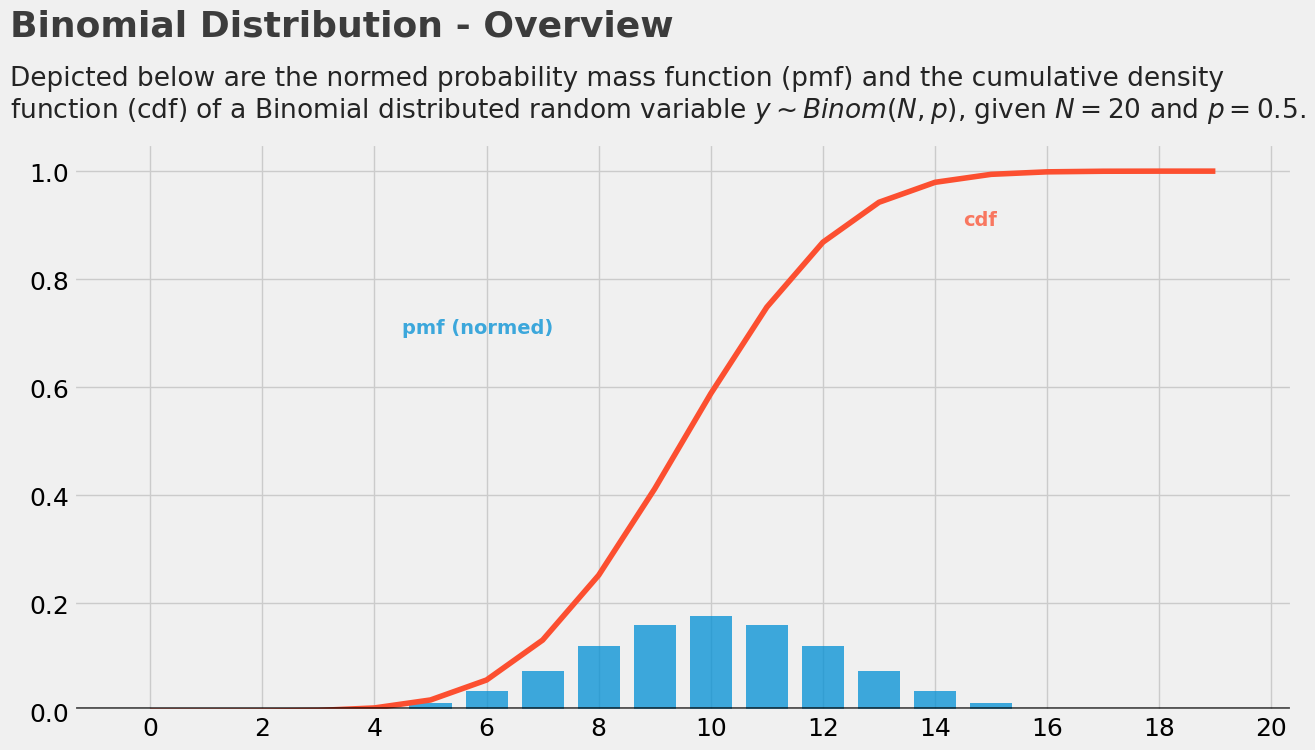改变P值后，对结果的影响如下图所示：

plt.figure(dpi=100)

# PDF P = .2
plt.scatter(np.arange(21),
(stats.binom.pmf(np.arange(21), p=.2, n=20)),
alpha=0.75,
s=100
)
plt.plot(np.arange(21),
(stats.binom.pmf(np.arange(21), p=.2, n=20)),
alpha=0.75,
)

# PDF P = .5
plt.scatter(np.arange(21),
(stats.binom.pmf(np.arange(21), p=.5, n=20)),
alpha=0.75,
s=100
)
plt.plot(np.arange(21),
(stats.binom.pmf(np.arange(21), p=.5, n=20)),
alpha=0.75,
)

# PDF P = .9
plt.scatter(np.arange(21),
(stats.binom.pmf(np.arange(21), p=.9, n=20)),
alpha=0.75,
s=100
)
plt.plot(np.arange(21),
(stats.binom.pmf(np.arange(21), p=.9, n=20)),
alpha=0.75,
)

# LEGEND
plt.text(x=3.5, y=.075, s="$p = 0.2$", alpha=.75, weight="bold", color="#008fd5")
plt.text(x=9.5, y=.075, s="$p = 0.5$", alpha=.75, weight="bold", color="#fc4f30")
plt.text(x=17.5, y=.075, s="$p = 0.9$", alpha=.75, weight="bold", color="#e5ae38")

# TICKS
plt.xticks(range(21)[::2])
plt.tick_params(axis = 'both', which = 'major', labelsize = 18)
plt.axhline(y = 0, color = 'black', linewidth = 1.3, alpha = .7)

# TITLE, SUBTITLE & FOOTER
plt.text(x = -2.5, y = .37, s = "Binomial Distribution - $p$",
fontsize = 26, weight = 'bold', alpha = .75)
plt.text(x = -2.5, y = .32,
s = 'Depicted below are three Binomial distributed random variables with varying $p$. As one can see\nthe parameter $p$ shifts and skews the distribution.',
fontsize = 19, alpha = .85)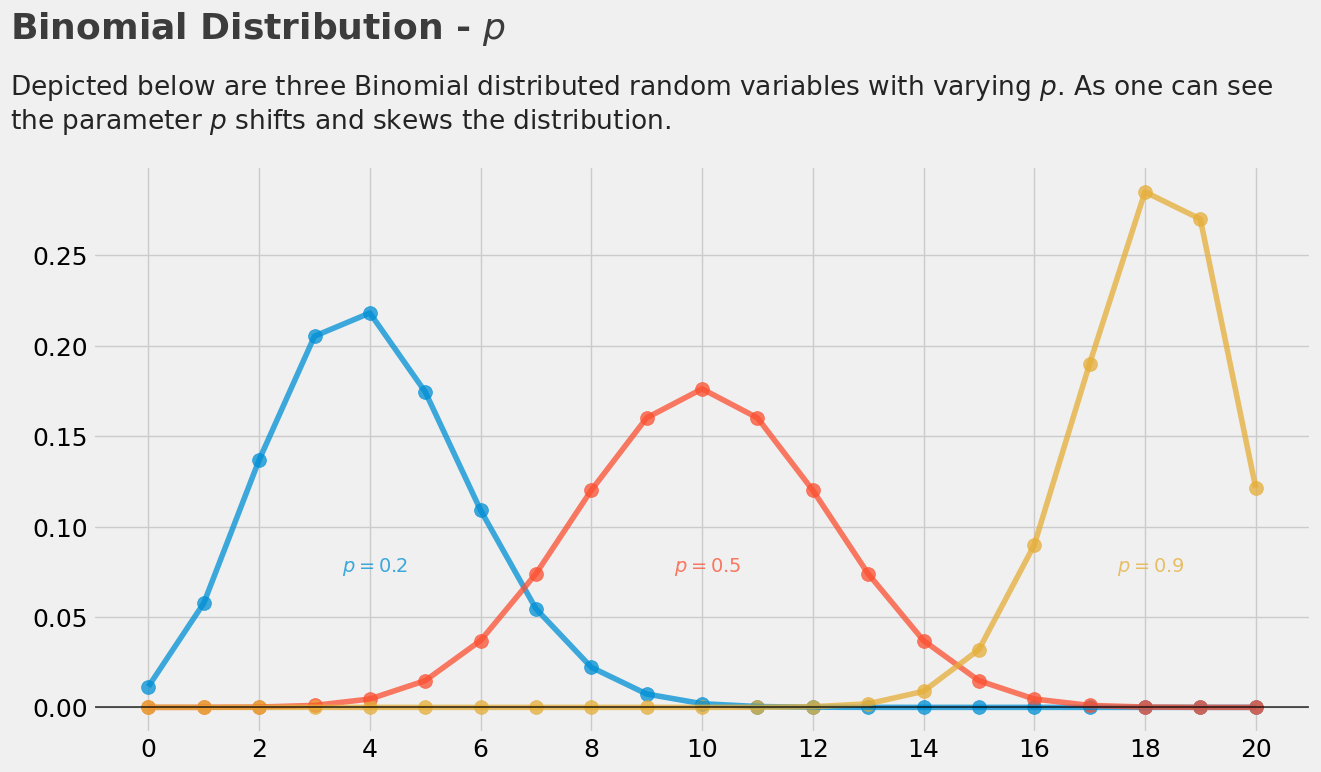改变N值对结果产生的影响如下所示：

plt.figure(dpi=100)

# PDF N = 10
plt.scatter(np.arange(11),
(stats.binom.pmf(np.arange(11), p=.5, n=10)),
alpha=0.75,
s=100
)
plt.plot(np.arange(11),
(stats.binom.pmf(np.arange(11), p=.5, n=10)),
alpha=0.75,
)

# PDF N = 15
plt.scatter(np.arange(16),
(stats.binom.pmf(np.arange(16), p=.5, n=15)),
alpha=0.75,
s=100
)
plt.plot(np.arange(16),
(stats.binom.pmf(np.arange(16), p=.5, n=15)),
alpha=0.75,
)

# PDF N = 20
plt.scatter(np.arange(21),
(stats.binom.pmf(np.arange(21), p=.5, n=20)),
alpha=0.75,
s=100
)
plt.plot(np.arange(21),
(stats.binom.pmf(np.arange(21), p=.5, n=20)),
alpha=0.75,
)

# LEGEND
plt.text(x=6, y=.225, s="$N = 10$", alpha=.75, weight="bold", color="#008fd5")
plt.text(x=8.5, y=.2, s="$N = 15$", alpha=.75, weight="bold", color="#fc4f30")
plt.text(x=11, y=.175, s="$N = 20$", alpha=.75, weight="bold", color="#e5ae38")

# TICKS
plt.xticks(range(21)[::2])
plt.tick_params(axis = 'both', which = 'major', labelsize = 18)
plt.axhline(y = 0, color = 'black', linewidth = 1.3, alpha = .7)

# TITLE, SUBTITLE & FOOTER
plt.text(x = -2.5, y = .31, s = "Binomial Distribution - $N$",
fontsize = 26, weight = 'bold', alpha = .75)
plt.text(x = -2.5, y = .27,
s = 'Depicted below are three Binomial distributed random variables with varying $N$. As one can see\nthe parameter $N$ streches the distribution (the larger $N$ the flatter the distribution).',
fontsize = 19, alpha = .85)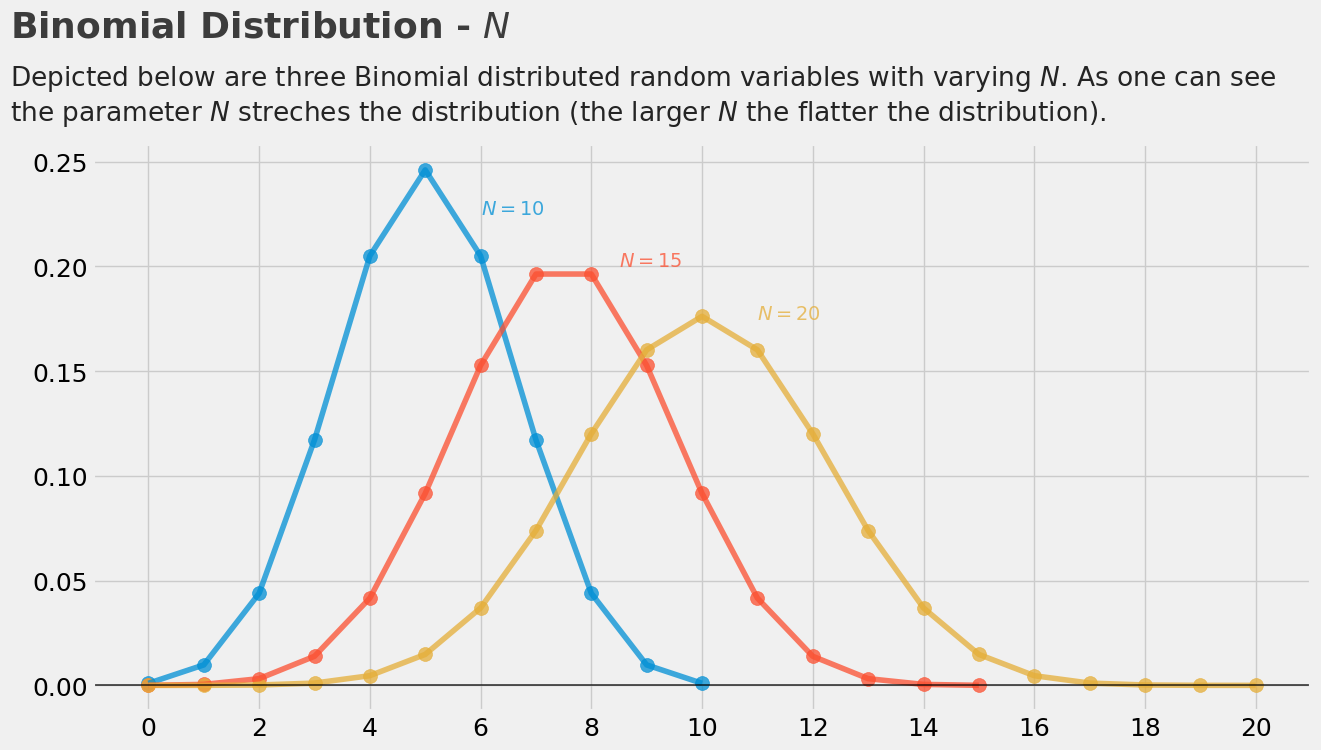也可以构造随机样本（Random Variates）：

import numpy as np
from scipy.stats import binom

# draw a single sample
np.random.seed(42)
print(binom.rvs(p=0.3, n=10), end="\n\n")

# draw 10 samples
print(binom.rvs(p=0.3, n=10, size=10), end="\n\n")
2

[5 4 3 2 2 1 5 3 4 0]

计算概率质量函数的概率：

from scipy.stats import binom

# additional imports for plotting purpose
import numpy as np
import matplotlib.pyplot as plt
%matplotlib inline
plt.rcParams["figure.figsize"] = (14,7)

# likelihood of x and y
x = 1
y = 7
print("pmf(X=1) = {}\npmf(X=7) = {}".format(binom.pmf(k=x, p=0.3, n=10), binom.pmf(k=y, p=0.3, n=10)))

# continuous pdf for the plot
x_s = np.arange(11)
y_s = binom.pmf(k=x_s, p=0.3, n=10)
plt.scatter(x_s, y_s, s=100);
pmf(X=1) = 0.12106082099999989
pmf(X=7) = 0.009001691999999992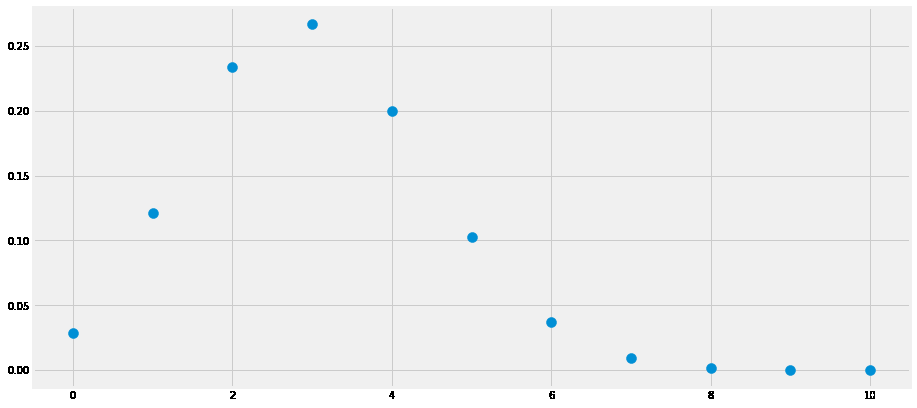计算累积概率密度函数的概率：

from scipy.stats import binom

# probability of x less or equal 0.3
print("P(X <=3) = {}".format(binom.cdf(k=3, p=0.3, n=10)))

# probability of x in [-0.2, +0.2]
print("P(2 < X <= 8) = {}".format(binom.cdf(k=8, p=0.3, n=10) - binom.cdf(k=2, p=0.3, n=10)))
P(X <=3) = 0.6496107183999998
P(2 < X <= 8) = 0.6170735276999999
展开全文python
• 系列文章目录 文章目录 系列文章目录 前言 一、操作步骤 二、主要结果 三、所有统计量和图形 四、选择特殊原因检验 五、minitab说明书解释参考 六、数据注意事项 总结 前言 使用二项式分布过程能力分析确定缺陷品...

# 前言

使用二项式分布过程能力分析确定缺陷品百分比是否符合客户的要求。当每个产品项都划分为两个类别中的一类（例如通过或未通过）时使用。使用此分析，可以执行以下操作：

• 确定过程是否受控制。
• 估计每个样本的缺陷品百分比，以及所有样本的缺陷品百分比（缺陷百分比）。
• 评估缺陷百分比是否稳定。

例如，某厂家分析良率是否满足客户要求的97.5%。

# 一、操作步骤

• 打开软件，打开样本数据；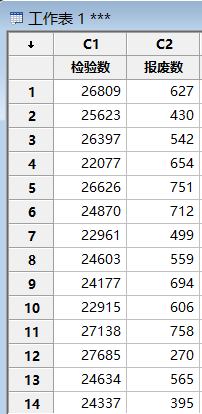• 选择统计>质量工具>能力分析>二项；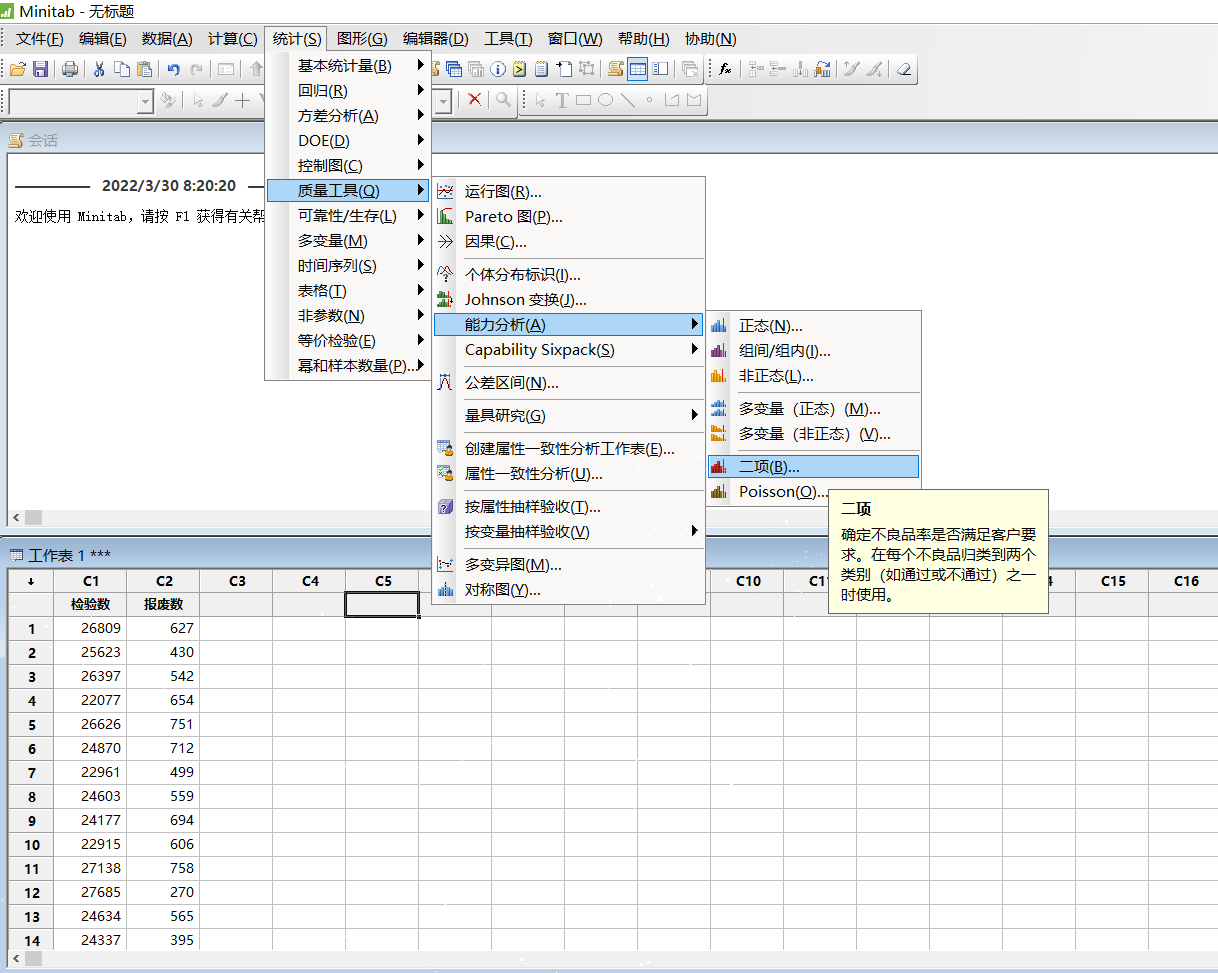• 在缺陷数中，输入报废数，在样本数量下，选择实际样本量(即检验数)，单击确认；
历史P值可选择预估的值，如果您知道总体分布中缺陷品的比率，或者如果您已从过去的数据中获得了过程估计值，请输入一个介于 0 到 1 之间的值以显示缺陷品历史比率。例如，如果缺陷品历史比率为 2.5％，则输入 0.025。如果不输入历史比率，Minitab 将从您的样本数据中估计比率，并使用估计值来计算过程能力。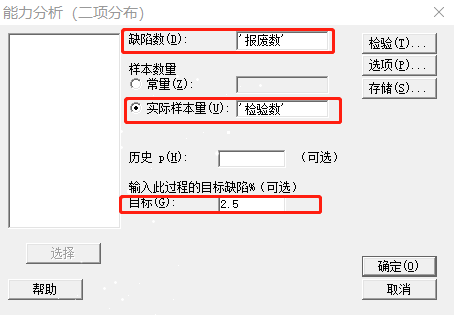• 得到二项过程能力报告；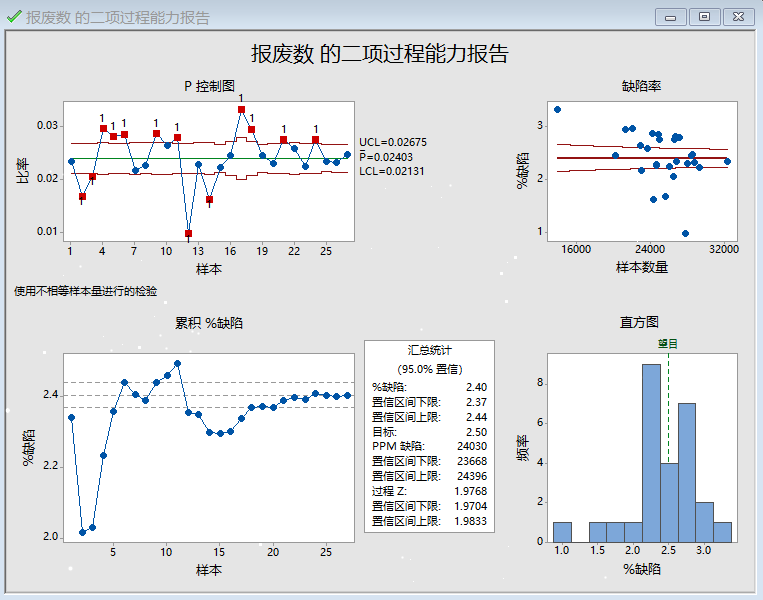• 要求缺陷为2.5%，现在缺陷为2.4%，PPM为24030；但是由于不满足二项分布原则，且样本选取不合适存在变异，因此结果不准确；

# 二、主要结果

• 步骤 1：确定您的过程是否稳定
• 步骤 2：确定数据是否服从二项分布
• 步骤 3：评估缺陷单元的百分比

步骤一：
在评估过程的能力之前，确定过程是否稳定。如果过程不稳定，则估计的过程能力可能不可靠。

可使用 P 控制图在视觉上监视缺陷百分比，以及确定缺陷百分比是否稳定并受控制。

红点表示至少未通过一个特殊原因检验且不受控制的子组。失控点表示过程可能不稳定，并且能力分析的结果可能不可靠。您应该在分析过程能力之前确定点失控的原因并消除特殊原因变异。

在此上面P 控制图中，一部分点随机变化，且在控制限之内。未显示出任何趋势或模式。但是，也有部分缺陷单位比率不受控制。在评估过程能力之前，需调查和消除可能于当日导致异常高的缺陷率的任何特殊原因。

步骤二：

在评估过程的能力之前，确定它是否服从二项分布。如果数据不服从二项分布，则估计的过程能力可能不可靠。Minitab 所显示的用于评估数据分布情况的图形取决于子组大小是否相同。

子组大小相同

如果所有子组大小相同，Minitab 会显示二项图。

检查此图以确定绘制点是否大致遵循一条直线分布。如果不是，那么“数据是从二项分布采样的”这一假定可能是错误的。

二项分布
在此图中，数据点紧密地分布在这条线的周围。您可以假设数据服从二项分布。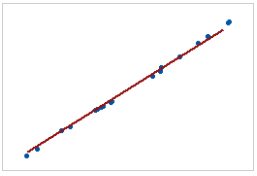非二项分布
在此图中，数据点不沿右上部附近的线分布。这些数据不服从二项分布，并且无法使用二项能力分析对其进行可靠评估。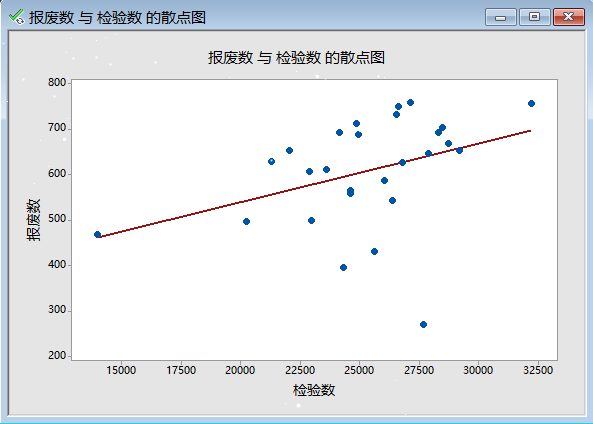子组大小不同

如果子组大小不同，Minitab 会显示缺陷率图。

检查此图以查看缺陷品百分比是否在整个样本数量中随机分布，或是否存在一种模式。如果数据随机分布在中心线周围，则可以推断数据服从二项分布。

二项分布
在此图中，点随机分布在中心线周围。您可以假设数据服从二项分布。因此，可使用二项能力分析评估数据。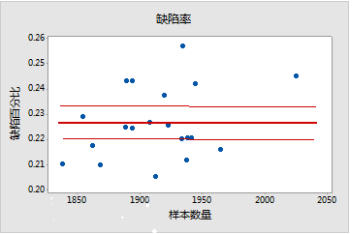非二项分布
在此图中，模式不是随机的。此结果表明在样本数量和缺陷百分比之间存在相关性。因此，这些数据不服从二项分布，并且无法使用二项能力分析对其进行可靠评估。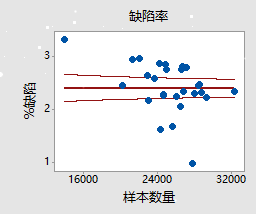步骤三：
检查缺陷百分比估计值和置信区间

使用样本数据的缺陷百分比均值来估计过程的缺陷百分比均值。使用置信区间作为估计值的误差容限。

置信区间提供了一系列您过程中缺陷百分比实际值的可能值（如果您能够收集和分析其生成的所有项）。在置信水平为 95％ 的情况下，您可以 95％ 地确信过程的实际缺陷百分比包含在置信区间内。也就是说，如果从过程中收集 100 个随机样本，可以期望约 95 个样本生成含有缺陷百分比实际值的区间。

置信区间有助于评估样本估计值的实际意义。如果您有一个基于过程知识或行业标准的最大允许缺陷百分比值，则将置信上限与该值进行比较。如果置信上限小于最大允许缺陷百分比值，则您可以确信过程符合规格，即使考虑到将影响估计值的随机抽样变异也是如此。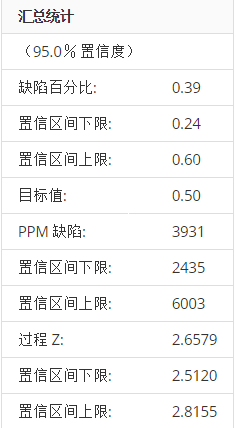主要结果：缺陷百分比，CI
二项能力分析的结果包括汇总统计表，其位于输出内容中间偏下部分。在此模拟的汇总统计表中，目标值 (0.50%) 表示过程的最大允许缺陷百分比。缺陷百分比估计值为 0.39％，其低于最大允许缺陷百分比值。但是，缺陷百分比的置信区间上限是 0.60％，其超出了最大允许值。因此，您无法 95％ 地确信此过程有能力。您可能需要使用更大的样本数量或者减少过程变异，以获得针对缺陷百分比估计值的更窄置信区间。

确定您是否具有足够数据以进行可靠的估计
可使用累积百分比缺陷图确定是否具有足够样本，从而能够获得百分比缺陷的稳定估计值。

检查按时间排序的缺陷品百分比，以查看当收集更多样本时估计值如何变化。理想情况下，缺陷品百分比在几个样本后稳定，由沿缺陷品均值线的变平绘制点显示。
样本足够
在此图中，缺陷百分比沿缺陷百分比均值线稳定地分布。因此，此能力研究包含了足够样本来稳定、可靠地估计缺陷百分比均值。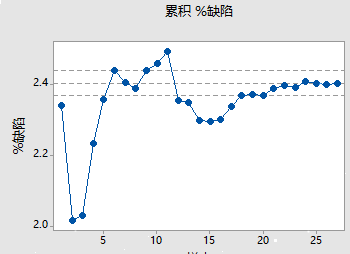样本不够
在此图中，缺陷百分比不稳定。因此，此能力研究未包含足够的样本来可靠地估计缺陷百分比均值。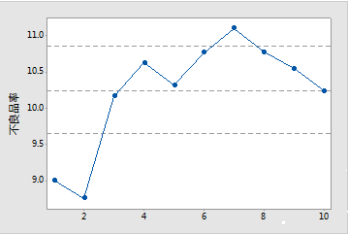# 三、所有统计量和图形

P 控制图
P 控制图绘制每个子组的不合格单元（也称缺陷品）的比率。中心线是所有子组缺陷品平均比率。控制限（设定为位于中心线上方和下方 3 个标准差的距离）显示子组比率中的预期变异量。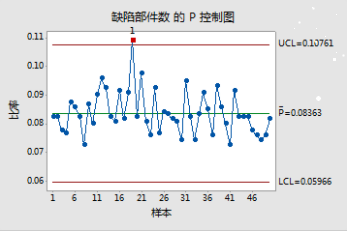此 P 控制图显示，平均而言，任一天的产品缺陷率为 8％。第 19 天的缺陷产品比率失控，因为其对应的值不在预期变异限制范围内。

解释
可使用 P 控制图在视觉上监视缺陷百分比，以及确定缺陷百分比是否稳定并受控制。

红点表示至少未通过一个特殊原因检验且不受控制的子组。失控点表示过程可能不稳定，并且能力分析的结果可能不可靠。您应该在分析过程能力之前确定点失控的原因并消除特殊原因变异。

特殊原因检验
特殊原因检验评估每个控制图上的标绘点是否随机分布在控制限制之内。

解释
使用特殊原因检验，可以确定需要调查的观测值和您数据中的具体模式和趋势。每项检验特殊原因检验将检测您数据中的具体模式或者趋势，这将揭示过程不稳定性的不同方面。

1 个点，距离中心线超过 3 个西格玛
检验 1 识别与其他子组相比异常的子组。检验 1 是公认的、用于检测失控情况的必要检验。如果对过程中的较小偏移感兴趣，您可以使用检验 2 来补充检验 1，以便创建一个敏感度更高的控制图。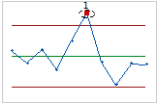连续 9 点在中心线同一侧
检验 2 会标识过程变异中的偏移。如果对过程中的较小偏移感兴趣，您可以使用检验 2 来补充检验 1，以便创建一个敏感度更高的控制图。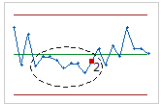连续 6 点，全部递增或全部递减
检验 3 检测趋势。该检验会查找值一致增加或减少的长序列连续点。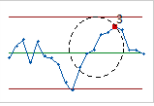连续 14 点上下交错
检验 4 检测系统变异。您希望过程中的变异模式随机，但是未通过检验 4 的点可能表示变异模式是可预测的。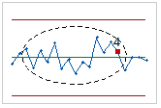累积缺陷百分比图
累积缺陷百分比图上的点表示每个样本的缺陷品百分比均值。这些点将按样本收集顺序显示。中间水平线代表从所有样本计算的缺陷品百分比均值。上部和下部水平线代表缺陷品百分比均值的置信区间上下限。

解释
可使用累积百分比缺陷图确定是否具有足够样本，从而能够获得百分比缺陷的稳定估计值。

检查按时间排序的缺陷品百分比，以查看当收集更多样本时估计值如何变化。理想情况下，缺陷品百分比在几个样本后稳定，由沿缺陷品均值线的变平绘制点显示。

样本足够
在此图中，缺陷百分比沿缺陷百分比均值线稳定分布。因此，该能力研究包含了足够样本来稳定、可靠地估计缺陷百分比均值。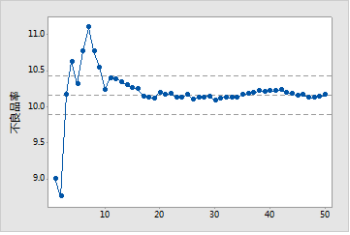样本不够
在此图中，缺陷百分比不稳定。因此，该能力研究未包含足够样本来可靠地估计缺陷百分比均值。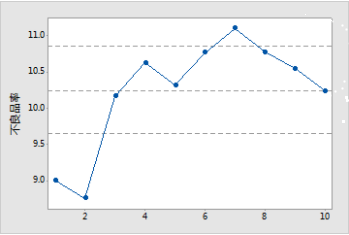二项图
二项图显示实测的缺陷品数与预期的缺陷品数。对角线显示完全服从二项分布时数据的分布位置。如果数据显著偏离这条线，则二项能力分析可能无法提供可靠结果。

注意
当子组大小相同时，Minitab 会显示二项图。如果子组大小不同，Minitab 会显示缺陷率图。有关详细信息，请参见关于缺陷率图的部分。

解释
可使用二项分布评估数据是否服从二项分布。

检查此图以确定绘制点是否大致遵循一条直线分布。如果不是，那么“数据是从二项分布采样的”这一假定可能是错误的。

在此图中，数据点紧密地分布在这条线的周围。您可以假设数据服从二项分布。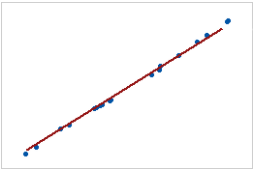在此图中，数据点不沿图右上部附近的线分布。这些数据不服从二项分布，并且无法使用二项能力分析对其进行可靠评估。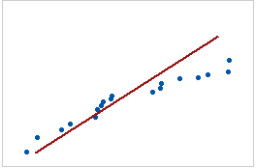重要信息
如果点不遵循线分布，则二项分布可能不适用于您的数据，而且能力分析可能会无效。

缺陷率图
缺陷品比率图显示子组中缺陷品的百分比以及每个子组的大小。中心线等于有缺陷的产品的平均概率。均值的置信上下限显示在中心线的上方和下方。

注意
Minitab 会在子组大小不同时显示缺陷率图。如果子组大小是恒定的，则 Minitab 会显示二项图。有关详细信息，请参见关于二项图的部分。

解释
使用缺陷品率图验证您的数据是否为二项数据，方法是检查缺陷品概率是否在不同样本数量之间保持不变。

检查此图以查看缺陷品百分比是否在整个样本数量中随机分布，或是否存在一种模式。如果数据随机分布在中心线周围，则可以推断数据服从二项分布。

二项
在此图中，点随机分布在中心线周围。您可以假设数据服从二项分布。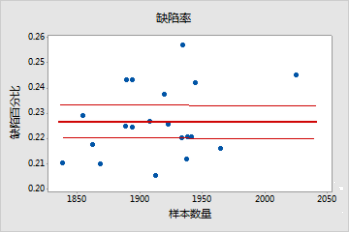非二项分布
在此图中，模式不是随机的。对于大于 1900 的样本数量，缺陷率随样本数量增加而增高。此结果表明在样本数量和缺陷百分比之间存在相关性。因此，数据不服从二项分布，并且无法使用二项能力分析对其进行可靠估计。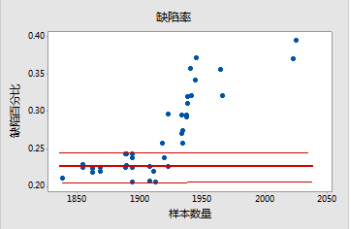直方图
此直方图显示样本中缺陷品百分比的分布。这些条形显示每个区间内缺陷品百分比的频率。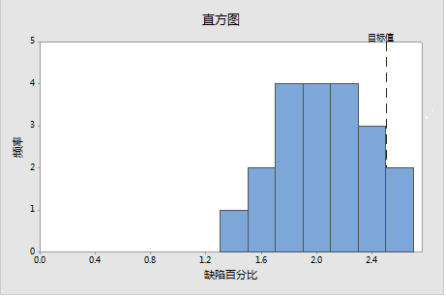解释
可使用缺陷百分比直方图评估样本中缺陷百分比值的分布。

检查缺陷品百分比的峰值和展开。峰值表示最普通的值，并接近于缺陷品百分比的中心。评估展开以了解样本中缺陷品百分比的变化程度。

将目标值的基准线与直方图的条形进行比较。如果过程有能力，则大多数或所有直方图条形应在目标值的左侧。

缺陷百分比
缺陷品百分比（%缺陷品）是样本中无法接受的产品百分比。其他产品可分类为“合格”或“良好”。

解释
可使用缺陷百分比确定您的过程是否满足客户要求。

将缺陷品百分比值与目标缺陷品百分比值进行比较，以评估过程是否满足客户要求。如果缺陷品百分比值比目标值高，应改进过程。

还应将目标值与缺陷品百分比的置信区间上限进行比较。如果置信区间上限大于目标值，您将无法确信过程的缺陷品百分比低于目标。您可能需要更大的样本数量以更有信心地确定过程达到目标。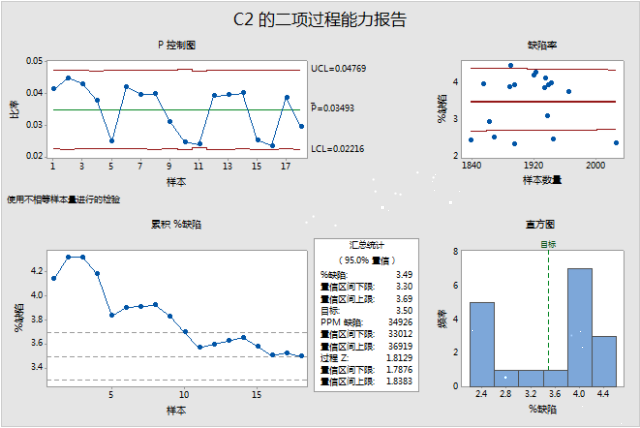例如，假设客户服务过程的缺陷百分比不应超过 3.5％。在汇总统计表中，缺陷百分比是 3.49％，其小于目标值。但是，缺陷百分比的置信区间上限是 3.69％，其大于目标值。虽然缺陷百分比的样本估计值低于目标值，但是您需要更大的样本数量以便更有信心地确定过程的缺陷百分比是否满足客户要求。

目标值
目标缺陷品百分比值是您愿意接受的最大缺陷品百分比值。如果不指定此目标值，Minitab 将假定目标值是缺陷率百分比 0。

解释
将缺陷品百分比值与目标缺陷品百分比值进行比较，以评估过程是否满足客户要求。如果缺陷品百分比值比目标值高，应改进过程。

还应将目标值与缺陷品百分比的置信区间上限进行比较。如果置信区间上限大于目标值，您将无法确信过程的缺陷品百分比低于目标。您可能需要更大的样本数量以更有信心地确定过程达到目标。

例如，在汇总统计表中，缺陷百分比值是 3.46％，其小于目值标 (3.50％)。但是，缺陷百分比的置信区间上限是 3.66％，其大于目标值。虽然过程似乎满足要求，但是您需要更大的样本数量以便更有信心地确定缺陷百分比值是否低于目标值。PPM 缺陷
缺陷品百万分数（缺陷品 PPM）估计预期的缺陷品百万分数。如果您从当前过程收集 1,000,000 个产品样本，缺陷品 PPM 是样品中的缺陷品的大致数量。

解释
将 PPM 缺陷值与客户要求进行比较，以确定您的过程是否需要改进。

还应考虑 PPM 缺陷的置信区间上限。如果置信区间上限大于允许的最大值，则无法确信过程满足客户要求。您可能需要更大的样本数量以更有信心地确定过程满足客户要求。例如，在汇总统计表中，PPM 缺陷值是 34,926。如果客户要求 PPM 缺陷值低于 35,000，则该过程符合要求。但是，置信区间上限是 36,919，其大于客户的要求值。因此，您需要更大的样本数量以便更有信心地确定过程是否可接受。

过程 Z
过程 Z 是标准正态分布 N (0, 1) 中其右侧面积等于 P 均值（过程中有缺陷品的比率）的点。

过程 Z 值 0 对应于缺陷品率 50％。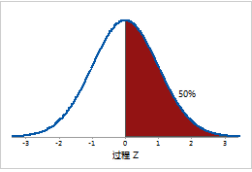过程 Z 值 2 对应于缺陷品率 2.275％。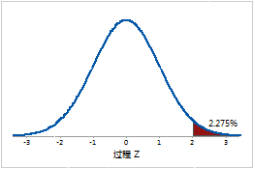解释
可使用过程 Z 评估二元过程的西格玛能力。

较大的 Z 值表明过程执行得较好。理想情况下，过程 Z 值至少为 2。过程的目标值取决于您客户的缺陷品的后果。

置信区间 (CI)
置信区间是能力指数的可能值范围。置信区间由置信下限和置信上限定义。边界是通过确定样本估计值的边际误差来计算的。置信下限定义可能小于能力指数值的值。置信上限定义可能大于能力指数值的值。

Minitab 同时显示缺陷百分比、PPM 缺陷和过程 Z 的置信下限和置信上限。

解释
由于数据样本是随机的，因此从过程收集的不同样本不可能生成相同的能力指数估计值。要计算过程能力指数的实际值，您将需要分析由过程生成的所有项数据，而这是不可行的。您可以改为使用置信区间来确定能力指数值的可能范围。

在 95% 置信水平下，您可以 95% 地确信能力指数的实际值包含在置信区间内。也就是说，如果您从过程中收集 100 个随机样本，则可以预计 95 个样本生成的区间中包含能力指数的实际值。

置信区间有助于评估样本估计值的实际意义。如果可能，基于过程知识或行业标准将置信边界与基准值进行比较。

例如，某制造过程的允许最大缺陷比率是 0.50％。通过使用二项式分布过程能力分析，分析师获得缺陷百分比估计值 0.31％，这表明该过程有能力。缺陷百分比的置信区间上限为 0.48%。因此，分析师可以 95％ 地确信缺陷百分比实际值不超过允许最大值，即使在考虑将会影响估值的随机抽样中变异也是如此。

# 四、选择特殊原因检验

选择统计>质量工具>能力分析>二项>检验；

使用特殊原因检验，可以确定需要调查的观测值和您数据中的具体模式和趋势。Minitab 提供了四种不同的特殊原因检验。请根据公司或行业标准选择其他检验。

在下拉列表中，指定是执行部分特殊原因检验、全部特殊原因检验还是不执行特殊原因检验。

1 个点，距离中心线超过 3 个西格玛
检验 1 识别与其他子组相比异常的子组。检验 1 是公认的、用于检测失控情况的必要检验。如果对过程中的较小偏移感兴趣，您可以使用检验 2 来补充检验 1，以便创建一个敏感度更高的控制图。
连续 9 点在中心线同一侧
检验 2 会标识过程变异中的偏移。如果对过程中的较小偏移感兴趣，您可以使用检验 2 来补充检验 1，以便创建一个敏感度更高的控制图。
连续 6 点，全部递增或全部递减
检验 3 检测趋势。该检验会查找值一致增加或减少的长序列连续点。
连续 14 点上下交错
检验 4 检测系统变异。您希望过程中的变异模式随机，但是未通过检验 4 的点可能表示变异模式是可预测的。

选择统计>质量工具>能力分析>二项>选项；

我们可以为过程能力报表输入自定义标题。

选择统计>质量工具>能力分析>二项>存储；
可以将分析统计量保存到工作表中，以便可以将它们用在其他分析、图形和宏中。Minitab 将选定的统计量存储在最后一个数据列后面。存储列的名称以一个数字结尾，如果您将同一个统计量存储多次，结尾的数字会递增。

有关其中任何统计量的更多详细信息，请转到所有统计量和图形。

变量名
存储用于分析的计数的列名称。Minitab 将该信息存储在 VarName1 列中。
P 的均值
存储过程中缺陷项的平均比率。Minitab 将该值存储在 AveP1 列中。
P 均值的下限
存储过程中缺陷项平均比率的置信下限。Minitab 将该值存储在 LBAveP1 列中。
P 均值的下限
存储过程中缺陷项平均比率的置信上限。Minitab 将该值存储在 UBAveP1 列中。
不良品率
存储样本中缺陷项的百分比。Minitab 将该值存储在 %Def1 列中。
不良品率的下限
存储过程中缺陷项百分比的置信下限。Minitab 将该值存储在 LB%Def1 中。
不良品率的上限
存储过程中缺陷项百分比的置信上限。Minitab 将该值存储在 UB%Def1 列中。
目标
存储过程的目标缺陷项百分比。Minitab 将该值存储在 Target1 列中。
PPM 缺陷
存储预期的每百万部件中缺陷部件的数量。Minitab 将该值存储在 PPMDef1 列中。
PPM 缺陷的下限
存储预期的每百万部件中缺陷部件数的置信下限。Minitab 将该值存储在 LBPPMDef1 列中。
PPM 缺陷的上限
存储预期的每百万部件中缺陷部件数的置信上限。Minitab 将该值存储在 UBPPMDef1 列中。
过程 Z
存储西格玛能力统计量过程 Z。Minitab 将该值存储在 ProcessZ1 列中。
过程 Z 的下限
存储西格玛能力统计量过程 Z 的置信下限。Minitab 将该值存储在 LBProcessZ1 列中。
过程 Z 的上限
存储西格玛能力统计量过程 Z 的置信上限。Minitab 将该值存储在 UBProcessZ1 列中。

# 五、minitab说明书解释参考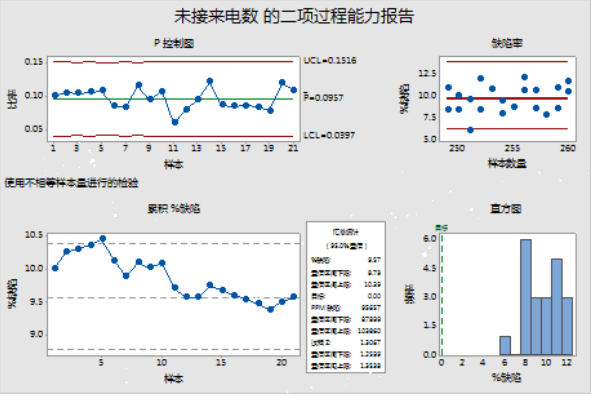对于缺陷率图，点似乎在各种样本数量上是随机分布的，因此管理员可以假定样本数量不影响缺陷率。P 控制图和累积缺陷百分比图表明此过程的缺陷百分比相当稳定。因此，似乎可满足能力分析的假设。

在汇总统计表中，缺陷百万分比（缺陷 PPM 值）表示每一百万个来电中预期有 95,657 个未接来电（缺陷）。此 PPM 值约对应于缺陷百分比 9.57％。置信区间 (CI) 上限和下限表明管理员可以 95％ 地自信过程缺陷百分比包含在区间 8.79％ 和 10.39％ 内。过程 Z 值 1.3 小于 2，这通常被视为能力过程所需的最低值。总的来说，这些摘要统计量表明呼叫中心不能满足规定要求。较高比例的呼叫都未被应答。管理员需要确定为何如此多的来电未接，以及如何改进过程。

# 六、数据注意事项

为了确保结果有效，请在收集数据、执行分析和解释结果时考虑以下准则。

数据必须为缺陷项的计数
子组中的每个项必须分类为可接受或不可接受（缺陷品）。如果数据不是缺陷品数，则无法使用二项分布来估计过程的能力。如果有针对每个项的缺陷数，请使用 Poisson 能力分析 评估每单位的缺陷数。
收集子组内数据
子组是相似项的集合，这些项是您要评估的过程的输出代表。应在相同的过程条件（如人员、设备、供应商或环境）下收集各子组的项。如果没有在相同的过程条件下收集子组中的数据，则子组中的变异可能会反映特殊原因变异，而非过程的自然、固有变异。

收集足够的子组以获取过程能力的可靠估计值
尝试收集至少 25 个子组。如果没有在足够长的一段时间内收集到充足的数据量，数据可能无法准确地代表不同的过程变异源，并且估计值可能无法指示过程的真实能力。
子组必须足够大
对于所有子组，平均缺陷品比率乘以子组大小应当至少为 .5。 如果子组大小不够大，则根据数据估计的控制限可能不可靠。
子组大小可以不同
子组大小可以存在差异。例如，如果某个呼叫中心每小时追踪 100 个来电，并对不满意等待时间的次数进行计数，则所有子组大小都为 100。但是，如果呼叫中心追踪一天中随机选定的小时内的所有打入的电话，来电数可能会不相同，从而产生不同的子组大小。子组大小可能存在差异。例如，如果一家呼叫中心每小时跟踪 100 通来电，并统计不满意等候时间的数量，则所有子组大小都为 100。但是，如果呼叫中心跟踪一天中随机选择的一小时内的所有来电，则来电数可能会不同，从而导致子组大小不相等。

过程必须稳定且受控制
如果当前过程不稳定，则将无法使用能力指标来可靠地评估将来的过程持续能力。 使用二项能力分析输出中的 P 控制图可确定过程是否稳定且受控制。 在评估过程能力前，请先调查失控点并消除过程中的所有特殊原因导致的变异。

# 总结

分享：
瞎看固然可以暂时满足自己的好奇心，但那种满足太暂时了，看过即忘，无法达成进一步的发展。现在让我写出几行量子力学的方程式来，对不起，一行也写不出来，但当时我是花了上千小时看的——这是把量子力学当美剧看，而且看的原因也是因为它很重要，觉得人活一生,连量子力学都没欣赏过,那真是太亏了。 ----石康

展开全文二项分布过程能力
• 二项式分布 二项式分布就是只有两个可能结果的分布，比如成功或失败，抛一枚硬币的正反面， 两个可能结果的概率可以相等，也可以是不相等的，总和为1，例如如果成功的概率为0.2，那么失败的概率就是P=1-0.2=0.8. 每...

## 二项式分布

二项式分布就是只有两个可能结果的分布，比如成功或失败，抛一枚硬币的正反面， 两个可能结果的概率可以相等，也可以是不相等的，总和为1，例如如果成功的概率为0.2，那么失败的概率就是P=1-0.2=0.8. 每一次尝试都是独立的，因为前一次投掷的结果不能影响当前投掷的结果，只有两种可能结果且重复n次的试验叫做二项式，二项式的参数是 n和P，n是试验的总次数，P是试验的概率。

在上述说明的基础上，二项式的属性包括： 1.每个试验都是独立的。 2.实验中只有两个可能的结果：成功和失败。 3.总共进行了相同地n次实验。 4.所有实验成功和失败的概率是相同的。（所有实验都是相同的）

公式：（写本上吧，太复杂打不出来）

PMF （概率质量函数）对离散随机变量的定义，是离散随机变量在各个特定取值的概率。该函数通俗来说，就是对一个离散型概率事件来说，使用该函数来求它各个成功事件结果的概率。 PDF (概率密度函数） 是对连续型随机变量的定义，与PMF不同的是，在特定点上的值并不是该点的概率，连续随机概率事件只能求连续一段区域内发生事件的概率，通过对这段区间进行积分，可获得事件发生时间落在给定间隔内的概率。

#二项式分布——画图
#导入需要的包
import numpy as np
import scipy.stats as stats
import matplotlib.pyplot as plt
import matplotlib.style as style
from IPython.core.display import HTML

#PLOTTING CONFIG 绘图配置
%matplotlib inline
style.use('fivethirtyeight')
plt.rcParams['figure.figsize']=(14,7)
plt.figure(dpi=100)

#PMF  绘制概率质量函数
plt.bar(left=(np.arange(20)),height=(stats.binom.pmf(np.arange(20),p=0.5,n=20)),width=0.75,alpha=0.75) #binom.pmf为二项式的概率质量数
#n=20,P=0.5,绘制成柱形图

#CDF
plt.plot(np.arange(20),stats.binom.cdf(np.arange(20),p=0.5,n=20),color="#fc4f30") #绘制该二项式的累积密度函数曲线

#LEGEND 图例
plt.text(x=7.5,y=0.2,s="pmf(binomed)",alpha=0.75,weight="bold",color="#008fd5")
plt.text(x=14.5,y=0.9,s="cdf",rotation=.75,weight="bold",color="#fc4f30")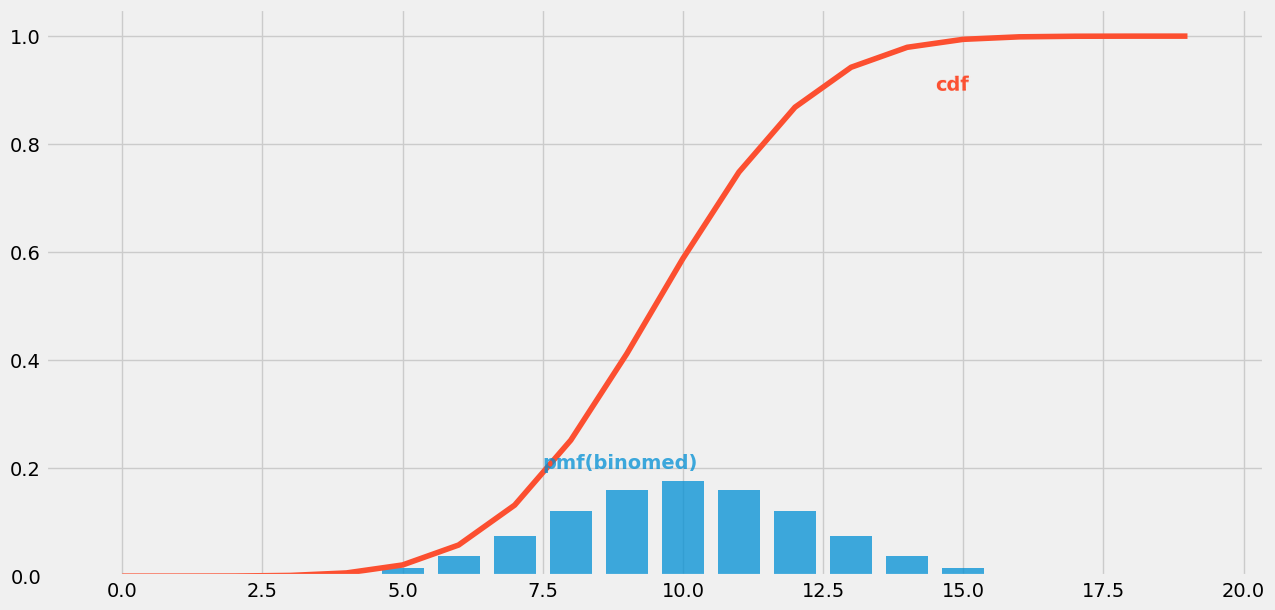P对结果的影响：

plt.figure(dpi=100)

#PDF P=0.2
plt.scatter(np.arange(21),stats.binom.pmf(np.arange(21),p=0.2,n=20),alpha=0.75,s=100)
plt.plot(np.arange(21),stats.binom.pmf(np.arange(21),p=0.2,n=20),alpha=0.75)

#PDF P=0.5
plt.scatter(np.arange(21),stats.binom.pmf(np.arange(21),p=0.5,n=20),alpha=0.75,s=100)
plt.plot(np.arange(21),stats.binom.pmf(np.arange(21),p=0.5,n=20),alpha=0.75)

#PDF P=0.9
plt.scatter(np.arange(21),stats.binom.pmf(np.arange(21),p=0.9,n=20),alpha=0.75,s=100)
plt.plot(np.arange(21),stats.binom.pmf(np.arange(21),p=0.9,n=20),alpha=0.75)

#LEGEND 图例
plt.text(x=3.5,y=0.075,s="#p=0.2#",alpha=0.75,weight="bold",color="#008fd5")
plt.text(x=9.5,y=0.075,s="#p=0.5",rotation=.75,weight="bold",color="#fc4f30")
plt.text(x=17.5,y=0.075,s="#p=0.9",rotation=.75,weight="bold",color="#fc4f30")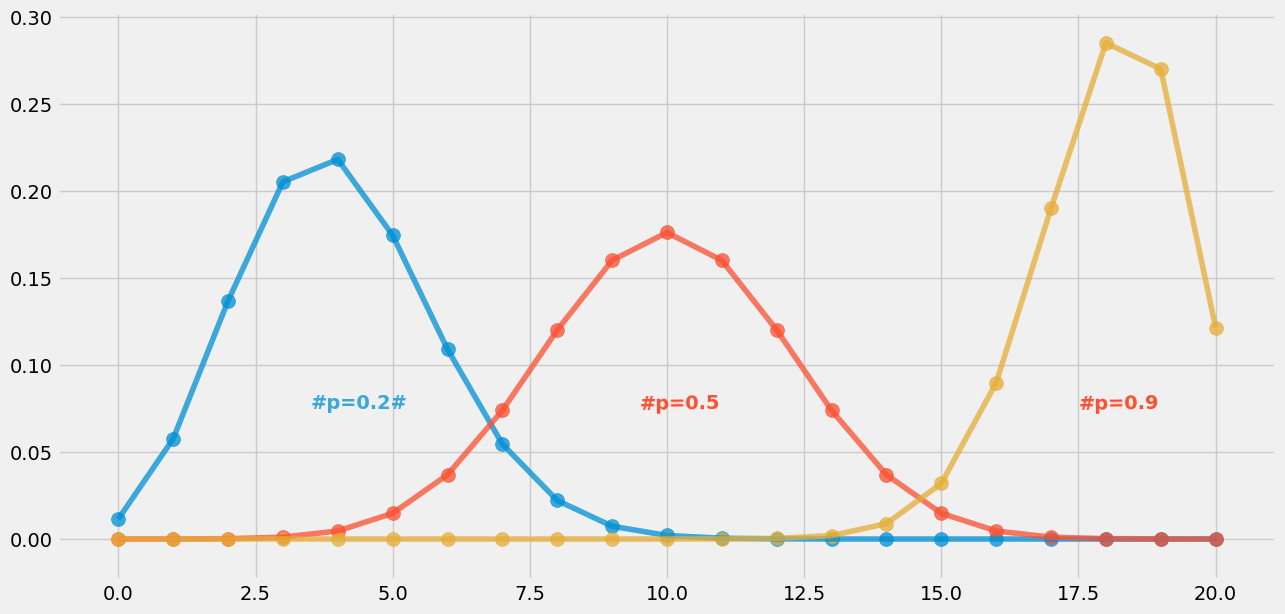当P不同时，赢m次的可能性的最大值都出现在均值处，对应概率为n*p。

N对结果的影响：

plt.figure(dpi=100)

#PDF N=10
plt.scatter(np.arange(21),stats.binom.pmf(np.arange(21),p=0.5,n=10),alpha=0.75,s=100)
plt.plot(np.arange(21),stats.binom.pmf(np.arange(21),p=0.5,n=10),alpha=0.75)

#PDF N=15
plt.scatter(np.arange(21),stats.binom.pmf(np.arange(21),p=0.5,n=15),alpha=0.75,s=100)
plt.plot(np.arange(21),stats.binom.pmf(np.arange(21),p=0.5,n=15),alpha=0.75)

#PDF N=20
plt.scatter(np.arange(21),stats.binom.pmf(np.arange(21),p=0.5,n=20),alpha=0.75,s=100)
plt.plot(np.arange(21),stats.binom.pmf(np.arange(21),p=0.5,n=20),alpha=0.75)

#LEGEND 图例
plt.text(x=6,y=0.25,s="$N=0.2$",alpha=0.75,weight="bold",color="#008fd5")
plt.text(x=8,y=0.2,s="$N=0.5$",rotation=.75,weight="bold",color="#fc4f30")
plt.text(x=10,y=0.175,s="$N=0.9$",rotation=.75,weight="bold",color="#fc4f30")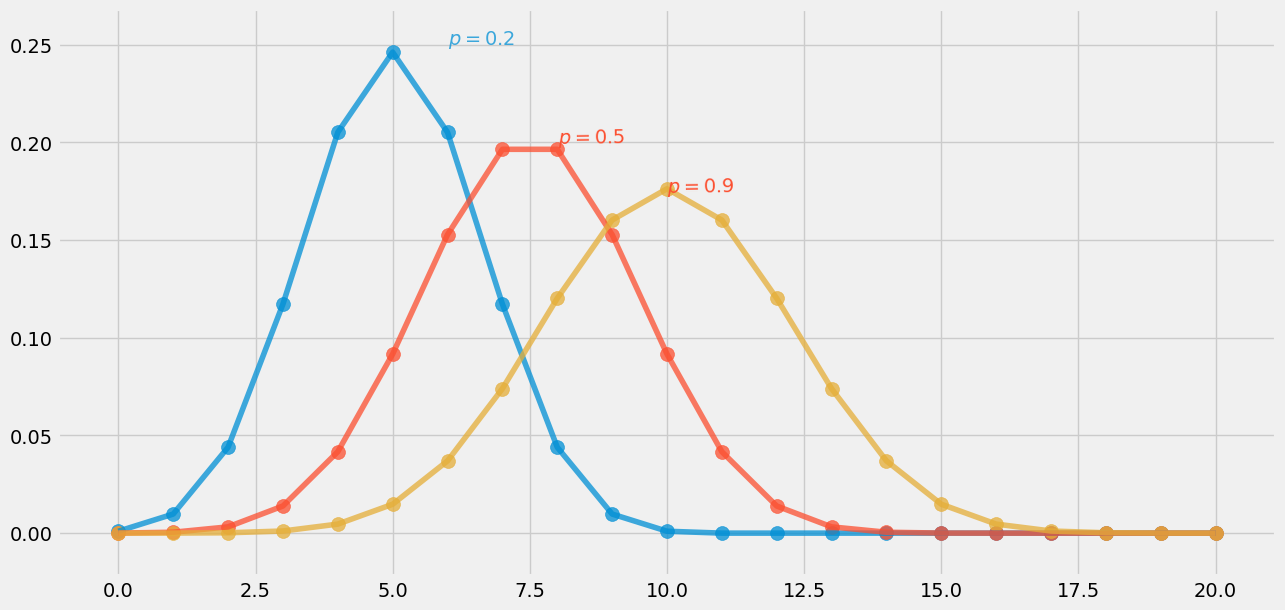当N不同时，成功m次的可能性的最大值都出现在均值处，对应概率为n*p。

#绘制PMF

import numpy as np
import scipy.stats as stats
import matplotlib.pyplot as plt
%matplotlib inline
plt.rcParams['figure.figsize']=(14,7)
#随机变量x、y的概率
x=1
y=7
print("p(x=1)={}\np(x=7)={}".format(stats.binom.pmf(k=x,p=0.3,n=10),stats.binom.pmf(k=x,p=0.3,n=10)))

#绘制二项式PMF
x_s=np.arange(11)
y_s=stats.binom.pmf(k=x_s,p=0.3,n=10)
plt.scatter(x_s,y_s,s=100) #离散型随机变量用散点图

p(x=1)=0.12106082100000018
p(x=7)=0.12106082100000018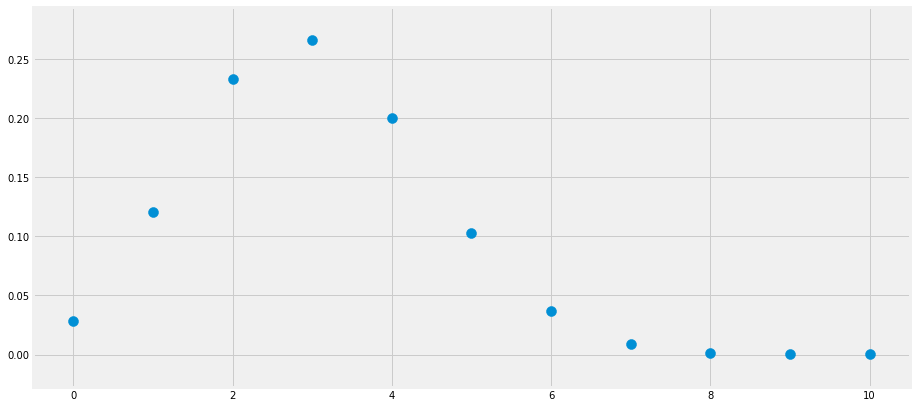#统计累积概率
#CDF
from scipy.stats import binom

#求成功小于三次的概率
print("p(x<=3) = {}".format(binom.cdf(k=3,p=0.3,n=10)))

#求成功次数大于2次，小于8次的概率
print("p(2< x <=8)={}".format(binom.cdf(k=8,p=0.3,n=10)- binom.cdf(k=2,p=0.3,n=10)))

展开全文python统计分析
• 二项分布二项分布是伯努利分布的推广，它模拟了在指定（非随机）失败次数（表示为r）发生之前，一系列独立且同分布的伯努利试验中的成功次数负二项分布
• (好久没写知乎文章了，又不知道该写什么，就随便水一水吧)二项分布： 次试验，每次试验有 的概率出现目标事件，记 为 次试验后出现目标事件的次数；负二项分布：若干次试验，每次试验有 的概率出现目标事件，记 为...
• 内容来自百度百科知识以及东方耀老师笔记内容的整合 1、独立重复试验的基本特征 ...2）每次试验都是只有两种结果：发生与不...7、二项式定理 参考资料 https://www.zhihu.com/question/38191693
• 知识点：伯努利分布、二项式分布、多项式分布、先验概率，后验概率，共轭分布、贝塔分布、贝塔-二项分布、负二项分布、狄里克雷分布，伽马函数、分布 一，伯努利分布(bernouli distribution) 又叫做0-1分布，...
• 1. 定义假设一串独立的伯努利实验（0-1，成功失败，伯努利实验），每次实验（trial）成功和失败的概率分别是 pp 和 1−p1-p。实验将会一直重复下去，直到实验失败了 rr 次。定义全部实验中成功的次数为随机变量 XX，...
• 指示器变量（Indicator variable）定义随机变量 xi∼b(1,μ)x_i\sim b(1, \mu)，xi,i=1,2,…,Nx_i, i=1,2,\ldots,N 彼此独立同分布，由相互独立的随机变量，以相互独立的随机变量 x,zx, z 为例，证明见 随机变量...
• ## 负指数二项式展开

千次阅读 2021-07-04 19:20:58
今日在学习陈希孺先生的《概率论与数理统计》一书时，看到了一个之前未闻的负二项分布，于是上溯到负指数的二项式展开，这方面从前未知，照例学习一番，写篇博客，即入即出。 含负数的组合数 我们通常的二项式展开的...
• 离散型变量 如：二项分布、泊松分布 三者之间的关系 二项分布(Binomial distribution) 二项分布(Binomial distribution)是n重伯努利试验成功次数的离散概率分布，记作。伯努利试验是只有两种可能结果的单次随机...二项分布 泊松分布
• https://blog.csdn.net/michael_r_chang/article/details/39188321https://www.cnblogs.com/wybang/p/3206719.htmlhttps://blog.csdn.net/jteng/article/details/603346281. 伯努利分布伯努利分布(Bernoulli ...伯努利分布 二项分布 多项分布 beta分布
• 二项分布是数学的概念，定义及公式定理不在此说明. 注：递归版第三步及递推都依赖于C（M-1，N-1）+C（M-1，N）=C（M，N）的变形 递归版: （我给递归版注释了1 2 3 是为了解析非递归版(递推版)中同等的操作 1 2 3...java 二项分布
• 如果无穷随机变量序列是独立同分布(i．i．d．)的，而且每个随机变量都服从参数为p的伯努利分布，那么随机变量就形成参数为p的一系列伯努利试验。同样，如果n个随机变量独立同分布，并且都服从参数为p的伯努利分布，...
• 说起二项分布(binomial distribution)，不得不提的前提是伯努利试验(Bernoulli experiment)，也即n次独立重复试验。伯努利试验是在同样的条件下重复、相互独立进行的一种随机试验。  伯努利试验的特点是： （1）...二项分布 伯努利分布
• 二项分布有两个参数，一个 n 表示试验次数，一个 p 表示一次试验成功概率。现在考虑一列二项分布，其中试验次数 n 无限增加，而 p 是 n 的函数。 1.如果 np 存在有限极限 λ，则这列二项分布就趋于参数为 λ 的 泊松...
• 伯努利分布（Bernoulli distribution）又名 两点分布 或 0-1分布，在讲伯努利分布前首先需要介绍伯努利试验（Bernoulli Trial）。 1.1 伯努利试验 伯努利试验是只有两种可能结果的单词随机试验，即对于一个随机变量 ...
• 二项式分布(binomial distrubution) 二项式分布：在n次试验中事件A恰好发生k次的概率。 概率计算： 式中k=0，1，2，…，n, 期望计算：E(x)=np 方差计算：D(x)=np(1-p) 例：抛n次硬币，正面朝上出现m次的概率。 ...机器学习 人工智能
• 二项分布是数学的概念，定义及公式定理不在此说明. 注：递归版第三步及递推都依赖于C（M-1，N-1）+C（M-1，N）=C（M，N）的变形 递归版: （我给递归版注释了1 2 3 是为了解析非递归版(递推版)中同等的操作 1 2 3...二项分布
• ## 二项分布

万次阅读 2013-12-11 09:58:48
二项分布[编辑] 维基百科，自由的百科全书 二项分布 机率 质量 函数 累积分布函数 参数  试验次数 (整数)  成功概率 (实数) 值域 概率密度函数 ...维基百科 二项分布
• 二项分布最大值，泊松分布的推导，几何分布的推导 (概统2.应用) 1.二项分布 二项分布就是独立事件n重伯努利试验，每次试验只有A发生与不发生两种结果，求n次试验中恰好发生k次的概率。 P{X=k} = \$C_{n}^{k}p^{k}q...二项分布 泊松分布 几何分布
• 1、泊松分布 由法国数学家西莫恩·德尼·泊松(Siméon-Denis Poisson)在1838年时发表； 若X服从参数为的泊松分布，记为X~P()， 泊松分布的概率分布函数： 参数λ是单位时间(或单位面积)内随机事件的平均发生率...
• 今天总结下二项分布、泊松分布和指数分布，直入主题吧： 先说说比较熟悉的二项分布吧，二项分布就是对同一实验重复n次，每次实验只有两个结果，设为事件A和事件B，N次实验中出现事件A的次数k的概率：可以看出这一...
• 伯努利分布二项分布、几何分布、超几何分布、泊松分布都是离散型随机变量。 1 伯努利分布：就是常见的0-1 分布，各自的频率为1-p和p ，当x=0 或者x=1 的时候，： p(x) = 期望： 方差： 对于伯努利分布来说 ，...
•   好久没写博客了，有好多都是写成了草稿没写完。列个清单慢慢补。。 数论专题。 概率\期望专题 划分树专题 ...搜出来是广义组合数，对应的有广义二项式定理。一看这个玩意儿ACM经常用就学一下。...
• 瑞士数学家雅克·伯努利(Jacques Bernoulli,1654～1705)首次研究独立重复试验(每次成功率为p)。在他去世后的第8年(1713年)，他侄子尼克拉斯出版了伯努利的著作...伯努利分布(Bernoulli distribution)又名两点分布或0机器学习 人工智能...